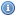# Matrix Books, Documents, and Lectures

 Title Year DescriptionAn Introduction to MatricesThese web pages are a Matrices tutorial and make a good "kick start" to the subject.Matrix Analysis and Applied Linear Algebra 2004The purpose of this text is to present the contemporary theory and applications of linear algebra to university students studying mathematics, engineering, or applied science at the postcalculus level.Matrix Tutorial 1: Stochastic Matrices 2006This tutorial introduces matrices, eigenvalues, and eigenvectors to IR students and search engine marketersMatrix Tutorial 2: Basic Matrix Operations 2006This tutorial covers matrix operations like addition, substractions, and multiplication of matrices. An introduction to multiplication and division of matrices by a scalar is provided. Includes determinants.The Matrix Cookbook 2006"The Matrix Cookbook" is a mathematical desktop reference on matrices.